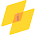## Search This Blog

Unit Impulse Response or Unit sample sequence:

The unit impulse signal is written as,

Unit Impulse Response or Unit sample sequence:
N=input('Enter the size of sequence=');
t=-N:N;
l=length(t);
for ii=1:l
if t(ii)==0
seq(ii)=1
else
seq(ii)=0
end
end
stem (t,seq);
title('Unit impulse Response');
xlabel('n');
ylabel('Amplitude');
Output waveform:
Enter the size of sequence=4

1.Try this code more simple:

N=input('Enter the size of sequence=');
t=-N:N;
seq=t==0;
stem(t,seq);
title('Unit Impulse Response');
xlabel('n');
ylabel('Amplitude');

1.Thanks for the program. Its working fine. I have posted your code https://www.matlabcoding.com/2020/04/unit-impulse-response-signal-generation.html

MATLAB